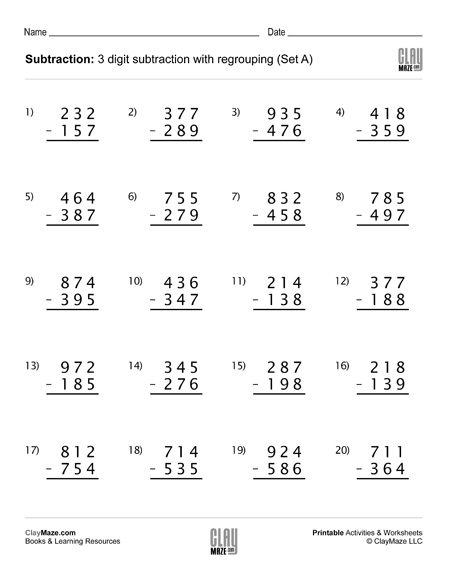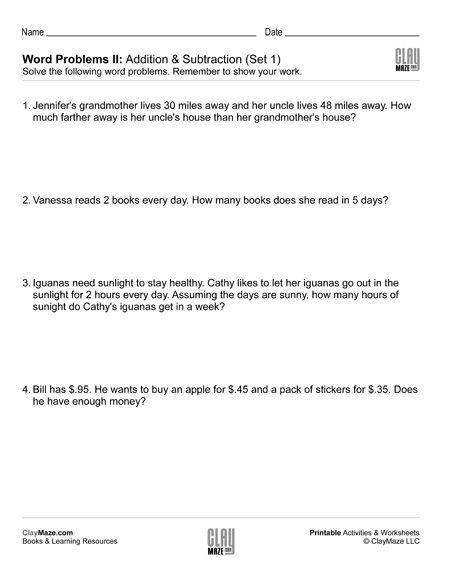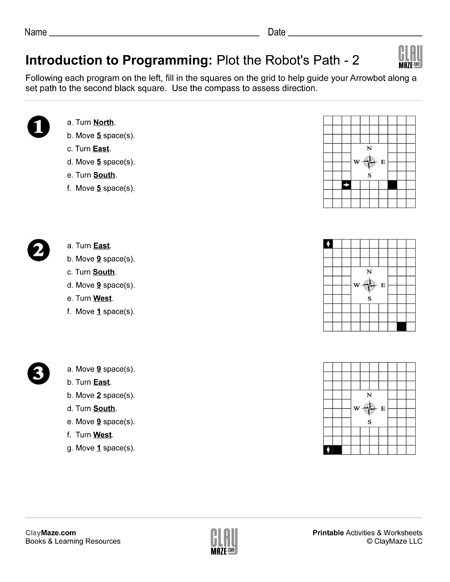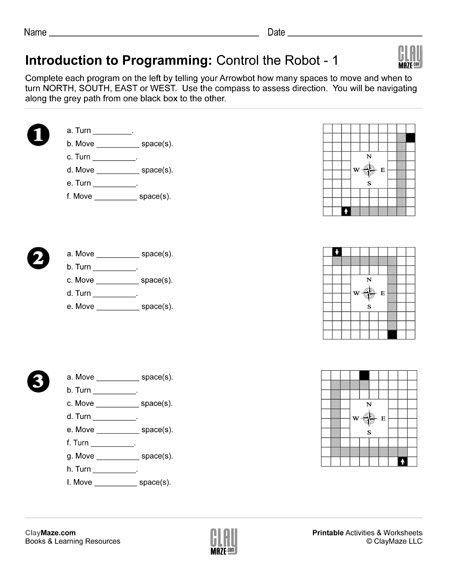Free Printable Worksheets for First Grade

## Subtraction Worksheet – 3 Digit Subtraction with Regrouping (Set D)3 digit subtraction worksheet with regrouping – 20 problems. There is extra spacing between the digits for borrowing....

## Subtraction Worksheet – 3 Digit Subtraction with Regrouping (Set C)Use this 3 digit subtraction worksheet with regrouping for lots of practice. There are 20 problems and numbers have enough spacing between the digits for borrowing....

## Subtraction Worksheet – 3 Digit Subtraction with Regrouping (Set B)Download this worksheet for subtraction practice with regrouping. The problems use 3 digit numbers with spacing between the digits to allow room for borrowing. There are 20 problems....

## Subtraction Worksheet – 3 Digit Subtraction with Regrouping (Set A)This worksheet contains 20 subtraction problems with regrouping. There is extra spacing between the digits for borrowing....

## Time Word Problems: Analog ClocksThese sets of worksheets are great for practice telling time with analog clocks and for understanding how to add and subtract with different time intervals. On these worksheets, the student is aske...

## Word Problems II: Addition & SubtractionThese sets of word problems are slightly more challenging than the Word Problems I sets. The student will need to use addition and subtraction to solve, but some problems use a little bit larger nu...

## Word Problems I: Addition & SubtractionThese are sets of basic addition and subtraction word problems. The student should read through each problem thoroughly to answer the questions and be sure to show their work below each one. These ...

## Introduction to Programming: Plot the Robot’s Path – 2Learning to interact with computers and equipment at an early edge is critical for today’s children. They are expected to interface and adapt to computers at an earlier and earlier age and any chan...

## Introduction to Programming: Plot the Robot’s Path – 1An early introduction to programming can give them the confidence they need and a great head start in STEM and computer related understanding.  This worksheet is part of a series on early...

## Introduction to Programming: Control the Robot – 2This worksheet is part of a set of 4 designed to introduce younger children to the very basics of computer programming.  The main series post is located here....

## Introduction to Programming: Control the Robot – 1STEM learning, or learning and preparation for careers in Science, Technology, Engineering and Mathematics has become a pervasive trend in today’s educational circles. With STEM occupations growing...

## Draw the Hands on the Analog Clocks (Set 4)Download this free worksheet for analog clock practice. Draw the hands on each of the clocks to match the times given. Don’t forget the movement of the hour hand as the minute hand goes around the ...